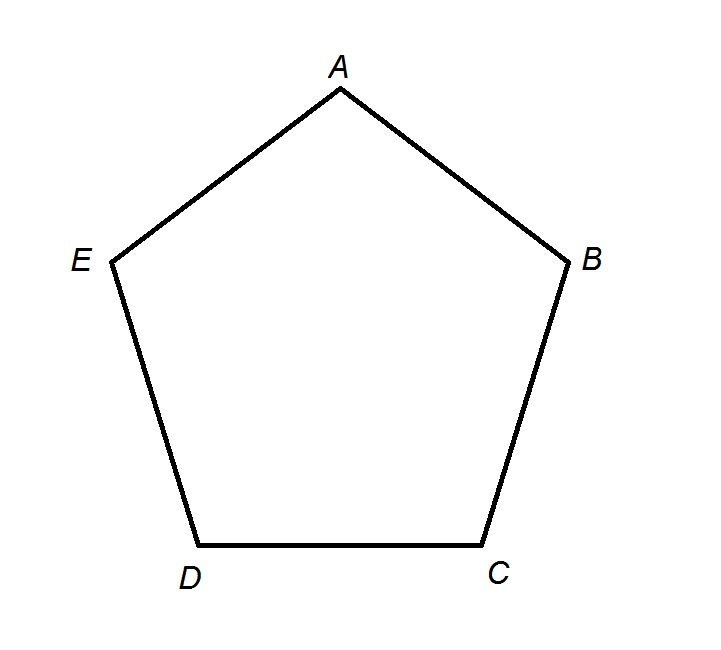# Want to know more about Pentagons?

Ah the Pentagon, part of the Polygon family. So you want to know more about Pentagons. You've seen this shape aroound but can't remember how many sides a Pentagon has. Here is how a Pentagon looks like.According to Dictionary.com, the Pentagon [pen-tuh-gon, -guhn] is a polygon having five angles and five sides. In the USA, Arlington, Virginia, the Pentagon was constructed to be an important place during the World War II. Being the headquarters of the USA Department of Defence, this has always been a symbol of leadership.1. What is a Pentagon? Pentagons can be regular or irregular and convex or concave. A regular pentagon is one with all equal sides and angles. Its interior angles are 108 degrees and its exterior angles are 72 degrees. An irregular pentagon is a shape that does not have equal sides and/or angles and therefore do not have specified angles.

2. What is an example of a Pentagon? There are examples of pentagons in real life in man-made structures like the Pentagon in the United States, and also in nature in flowers like morning glories and okra. Other items, like home plates in baseball, are often in the shape of irregular pentagons. A pentagon is a five-sided shape.

3. Is any 5 sided shape a Pentagon? In geometry, a pentagon (from the Greek πέντε pente and γωνία gonia, meaning five and angle) is any five-sided polygon or 5-gon. The sum of the internal angles in a simple pentagon is 540°. A pentagon may be simple or self-intersecting. A self-intersecting regular pentagon (or star pentagon) is called a pentagram.

4. Why Pentagon is a 2D shape. The word pentagon itself tells you what it is. ... A regular pentagon is one with all equal sides and angles. Its interior angles are 108 degrees and its exterior angles are 72 degrees. An irregular pentagon is a shape that does not have equal sides and/or angles and therefore do not have specified angles.

5. Can a Pentagon have curved sides? Circles and shapes that include curves are not polygons - a polygon, by definition, is made up of straight lines.

one cat
two cats
ten cats
no cats